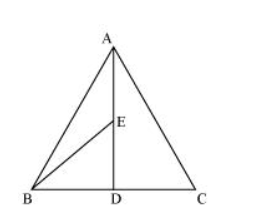# In a triangle ABC, E is the mid-point of median AD.

Question. In a triangle $A B C, E$ is the mid-point of median $A D .$ Show that $\operatorname{ar}(B E D)=\frac{1}{4} \operatorname{ar}(A B C)$

Solution:$\mathrm{AD}$ is the median of $\triangle \mathrm{ABC}$. Therefore, it will divide $\triangle \mathrm{ABC}$ into two triangles of equal areas.

$\therefore$ Area $(\triangle \mathrm{ABD})=$ Area $(\triangle \mathrm{ACD})$

$\Rightarrow$ Area $(\Delta A B D)=\frac{1}{2}$ Area $(\triangle A B C) \ldots$(1)

In $\triangle A B D, E$ is the mid-point of $A D$. Therefore, $B E$ is the median.

$\therefore$ Area $(\triangle B E D)=$ Area $(\triangle A B E)$

$\Rightarrow \operatorname{Area}(\Delta B E D)=\frac{1}{2}$ Area $(\triangle A B D)$

$\Rightarrow$ Area $(\Delta B E D)=\frac{1}{2} \times \frac{1}{2}$ Area $(\triangle A B C)$ [From equation (1)]

$\Rightarrow$ Area $(\Delta B E D)=\frac{1}{4}$ Area $(\triangle A B C)$